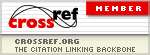### The Equity Premium and Inflation: Evidence from the US

Samih Antoine Azar

#### Abstract

There is recent and strong evidence that nominal stock returns are independent of inflation. In what amounts to the same thing, when real stock returns are regressed on inflation the resulting estimated coefficient on inflation is negative and unitary. These two propositions are mathematically equivalent. The purpose of this paper is to show that the market stock return is also independent of expected inflation, as measured by the T-bill rate. Firstly, regressions of the equity premium on inflation produce invariably slope coefficients that are statistically insignificantly different from -1. The inflation variable on the right hand side of the regression picks up the sign and the magnitude of the T-bill rate in the equity premium on the left hand side of the regression. This is as expected because the T-bill rate is an unbiased predictor of the future inflation rate, or, in other terms, the T-bill rate is a proxy for expected inflation. Hence regressions of real stock returns on inflation are in substance the same as regressions of the equity premium on inflation, and in both cases nominal stock returns are independent of inflation, and of its expected proxy, the T-bill rate. Secondly, additional evidence is provided from regressions of stock market returns on the inflation rate and the T-bill rate taken together. The hypothesis that the sum of the two coefficients on these two variables is statistically insignificantly different from zero is strongly supported. Moreover, the joint null hypothesis that the first coefficient is equal to -1 and that the second coefficient is equal to +1 is also strongly supported. As a conclusion stock prices already reflect macroeconomic shocks and there is neither money illusion nor over and under adjustment on the part of investors.

PDF

#### References

Azar, S. A. (2010). Inflation and stock returns, International Journal of Accounting and Finance, 2(3-4), 254-274. http://dx.doi.org/10.1504/IJAF.2010.034399.

Azar, S. A. (2014a). The Determinants of US Stock Market Returns, Open Economics and Management Journal, 1, 1-13. http://dx.doi.org/10.2174/2352630001401010001

Azar, S. A. (2014b). Inflation and stock returns II, International Journal of Economics and Finance, 6(1), 208-216. http://dx.doi.org/10.5539/ijef.v6n1p208

Basset, G. Jr., & Koenker, R. (1982). An empirical quantile function for linear models with i.i.d. errors, Journal of the American Statistical Association, 77(378), 407-415. http://dx.doi.org/10.1080/01621459.1982.10477826

Beirne, J., & de Bondt, g. (2008). The equity premium and inflation. Applied Financial Economics Letters, 4(6), 439-442. http://dx.doi.org/10.1080/17446540801935389

Bollerslev, T., & Wooldridge, J. M. (1992). Quasi-maximum likelihood estimation and inference in dynamic models with time varying covariances. Econometric Reviews, 11, 143-172. http://dx.doi.org/10.1080/07474939208800229

Fama, E., & French, K. (2002). The equity premium, Journal of Finance, 57(2), 637-659.

http://dx.doi.org/10.1111/1540-6261.00437

Frühwirth-Schnatter, S. (2006). Finite mixture and Markov switching models. New York: Springer Science + Business Media LLC.

Goetzmann, W. N., & Ibbotson, R. G. (2006). The equity risk premium, essays and explorations. New York: Oxford University Press.

Goldfeld, S. M., & Quandt, R. E. (`1973). A Markov model for switching regressions. Journal of Econometrics, 3-16. http://dx.doi.org/10.1016/0304-4076(73)90002-X

Goldfeld, S. M., & Quandt, R. E. (1976). Studies in nonlinear estimation. Cambridge, MA: Ballinger.

Hamilton, J. D. (1990). Analysis of time series subject to changes in regime. Journal of Econometrics, 45, 39-70. http://dx.doi.org/10.1016/0304-4076(90)90093-9

Hamilton, J. D. (1994). Time series analysis. Princeton: Princeton University Press.

Huber, P. J. (1973). Robust regression: asymptotics, conjectures and Monte Carlo, The Annals of Statistics, 1(5), 799-821. http://dx.doi.org/10.1214/aos/1176342503

Huber, P. J. (1981). Robust statistics. New York: John Wiley & Sons. http://dx.doi.org/10.1002/0471725250

Koenker, R. (1994). Confidence intervals for regression quantiles. In Asymptotic statistics, Mandl, P. & Huskova, H. (eds) New York: Springer-Verlag, 349-359. http://dx.doi.org/10.1007/978-3-642-57984-4_29

Koenker, R. (2005). Quantile regression. New York: Cambridge University Press.

http://dx.doi.org/10.1017/CBO9780511754098

Koenker, R., & Basset, G. Jr. (1978). Regression quantiles. Econometrica, 46(1), 33-50.

http://dx.doi.org/10.2307/1913643

Koenker, R., & Machado, J. A. F. (1999). Goodness of fit and related inference processes for quantile regression. Journal of the American Statistical Association, 94(448), 1296-1310.

http://dx.doi.org/10.1080/01621459.1999.10473882

Kyriacou, J. B., Madsen, J. B., & Mase, B. (2006). Does inflation exaggerate the equity premium? Journal of Economic Studies, 33(5), 344-356. http://dx.doi.org/10.1108/01443580610706573

Maddala, G. S. (1986). Disequilibrium, self-selection, and switching models, Handbook of econometrics, Griliches, Z. & Intriligator, M. D. (eds), Amsterdam: North-Holland.

Madsen, J. B., & Dzhumashev, R. (2009). The equity premium and the ex post bias, Applied Financial Economics, 19, 157-174. http://dx.doi.org/10.1080/09603100701765174

Mehra, R. (ed) (2008). Handbook of the equity risk premium. Amsterdam: Elsevier.

http://dx.doi.org/10.1016/B978-044450899-7.50006-3

Mehra, R., & Prescott, E. C. (1985). The equity premium: a puzzle. Journal of Monetary Economics, 15, 145-161. http://dx.doi.org/10.1016/0304-3932(85)90061-3

Nelson, D. B. (1991). Conditional heteroskedasticity in asset pricing: a new approach. Econometrica, 59, 347–370. http://dx.doi.org/10.2307/2938260

Newey, W. K., & West, K. D. (1987). A Simple, positive semi-deﬁnite, heteroskedasticity and autocorrelation-consistent covariance matrix. Econometrica, 55, 703–708. http://dx.doi.org/10.2307/1913610

Sharpe, S. A. (2002). Reexamining stock valuation and inflation: the implications of analysts' earnings forecasts. The Review of Economics and Statistics, 84(4), 632-648. http://dx.doi.org/10.1162/003465302760556468

Yaansah, R. A., & Pleasnell, K. V. (1994) Expectations, security yields, and inflation: ex-ante risk premia on UK shares, corporate bonds and gilts, 1969-1987, Journal of Business Finance & Accounting, 21(2), 155-174. http://dx.doi.org/10.1111/j.1468-5957.1994.tb00311.x

DOI: https://doi.org/10.11114/afa.v1i1.618

### Refbacks

• There are currently no refbacks.

Paper Submission E-mail: afa@redfame.com

Applied Finance and Accounting (AFA)

ISSN 2374-2410(Print)           ISSN 2374-2429(Online)